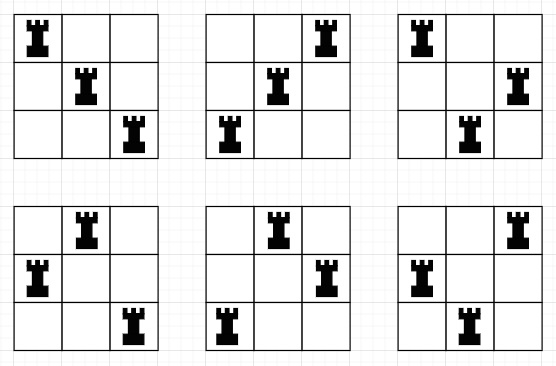# Program to find number of ways to arrange n rooks so that they cannot attack each other in Python

Suppose we have a number n representing a chessboard of size n x n. We have to find the number of ways we can place n rooks, such that they cannot attack each other. Here two ways will be considered different if in one of the ways, some cell of the chessboard is occupied, and another way, the cell is not occupied. (We know that rooks can attack each other if they are either on the same row or on the same column).

So, if the input is like 3, then the output will be 6To solve this, we will follow these steps −

f = factorial of n

return f

Let us see the following implementation to get better understanding −

## Example

Live Demo

import math
class Solution:
def solve(self, n):
return math.factorial(n)
ob = Solution()
print(ob.solve(3))

## Input

3

## Output

6